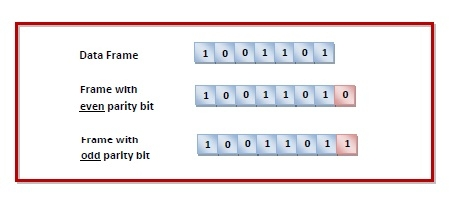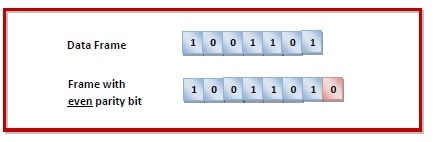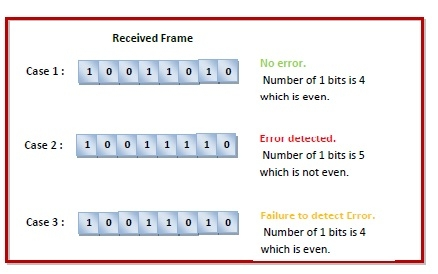# Error-Detecting Codes - Parity

## Errors and Error Detection

When bits are transmitted over the computer network, they are subject to get corrupted due to interference and network problems. The corrupted bits leads to spurious data being received by the receiver and are called errors.

Error detection techniques are responsible for checking whether an error has occurred or not in the frame that has been transmitted via the network. It does not take into account the number of error bits and the type of error.

For error detection, the sender needs to send some additional bits along with the data bits. The receiver performs necessary checks based upon the additional redundant bits. If it finds that the data is free from errors, it removes the redundant bits before passing the message to the upper layers.

There are three main techniques for detecting errors in data frames: Parity Check, Checksum and Cyclic Redundancy Check (CRC).

## Parity Bits

The parity check is done by adding an extra bit, called parity bit, to the data to make the number of 1s either even or odd depending upon the type of parity. The parity check is suitable for single bit error detection only.

The two types of parity checking are

• Even Parity  − Here the total number of bits in the message is made even.

• Odd Parity − Here the total number of bits in the message is made odd.

## Error Detection by Parity Check

Sender’s End − While creating a frame, the sender counts the number of 1s in it and adds the parity bit the value of which is determined as follows -

• In the case of even parity: If a number of 1s is even, the parity bit value is 0. If a number of 1s is odd, the parity bit value is 1.
•  In case of odd parity: If a number of 1s is odd, the parity bit value is 0. If a number of 1s is even, the parity bit value is 1.Receiver’s End − On receiving a frame, the receiver counts the number of 1s in it. In case of even parity check, if the count of 1s is even, the frame is accepted, otherwise, it is rejected. In case of odd parity check, if the count of 1s is odd, the frame is accepted, otherwise, it is rejected.

## Example

Suppose that a sender wants to send the data 1001101 using even parity check method. It will add the parity bit as shown below.The receiver will decide whether an error has occurred by counting whether the total number of 1s is even. When the above frame is received, three cases may occur namely, no error, single bit error detection and failure to detect multiple bits error. This is illustrated as follows -